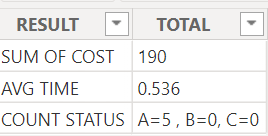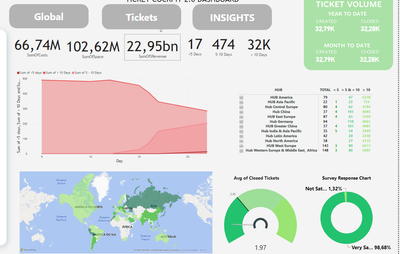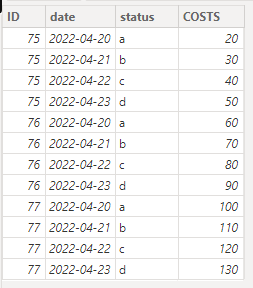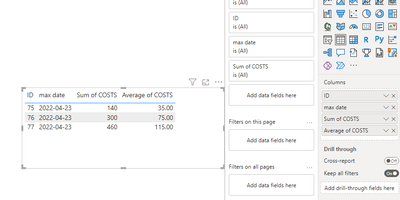cancel
Showing results for
Did you mean:Helper I

## Measures applied with DISTINCT ID based on the latest entry

Hello Community,

I have been struggling to make my dax measures in my current dashboard due to my data. I have my table called TICKET_DASHBOARD_HISTORICAL which contains different tickets indicated by the  ID column. Each ticket from the moment it has been created repeats itself every single day in my data. I have a DATE column which represents the day of the repetition of the ticket. So for example, if ticket number 75 was created on the 20/04/22 that ID will repeat everyday (21/22/23 and so on) and will keep repetiing for the rest of time. This is so we track the status of that ticket each day.

For all my measures where I will be calculating the SUM/AVERAGE and so on of a column I need to only consider the DISTINCT ticket and in most cases it would have to be the latest entry of that distinct ticket ID. So we would want to consider the latest DATE possible based on the DATE column.

I have some measures I tried to create based on my needs but it seems to not be working correctly:

SumOfCosts =
var a=SUMMARIZE(TICKET_DASHBOARD_HISTORICAL,TICKET_DASHBOARD_HISTORICAL[ID],TICKET_DASHBOARD_HISTORICAL[COSTS])
return SUM(TICKET_DASHBOARD_HISTORICAL[COSTS])

Count of Status =
VAR MaxDate =
IF(
COUNTROWS(ALLSELECTED('CALENDAR')) = 1,
CALCULATE(MAX(TICKET_DASHBOARD_HISTORICAL[Date])),
CALCULATE(
MAX(TICKET_DASHBOARD_HISTORICAL[Date]),
FILTER(
ALL(TICKET_DASHBOARD_HISTORICAL),
TICKET_DASHBOARD_HISTORICAL[Date] <= MAX(Calendar[Date])
)
)
)
VAR a =
CALCULATETABLE(
SUMMARIZE(
FILTER(
TICKET_DASHBOARD_HISTORICAL,
TICKET_DASHBOARD_HISTORICAL[Date] = MaxDate
),
TICKET_DASHBOARD_HISTORICAL[ID],
TICKET_DASHBOARD_HISTORICAL[Ticket Status]
),
ALLSELECTED('CALENDAR')
)
RETURN COUNTAX(a, TICKET_DASHBOARD_HISTORICAL[Ticket Status])

Here is my tabke structure:1 ACCEPTED SOLUTIONCommunity Support

We can create  two measures.

``````avg time1 =
var _date = CALCULATE(MAX('Table'[date]),FILTER(ALLSELECTED('Table'),'Table'[ID]=MAX('Table'[ID])))
return CALCULATE(AVERAGE('Table'[TICKET NAME]),FILTER(ALLSELECTED('Table'),'Table'[date]=_date))``````
``````sum of cost 1 =
var _date = CALCULATE(MAX('Table'[date]),FILTER('Table','Table'[ID]=MAX('Table'[ID])))
return CALCULATE(SUM('Table'[COSTS]),FILTER(ALLSELECTED('Table'),'Table'[date]=_date))``````

We can create  a calculated columns.

``````count status =
var _date = CALCULATE(MAX('Table'[date]),FILTER('Table','Table'[ID]=EARLIER('Table'[ID])))
var _a=CALCULATE(COUNT('Table'[status]),FILTER('Table','Table'[date]=_date && 'Table'[status]="A"))
var _b=CALCULATE(COUNT('Table'[status]),FILTER('Table','Table'[date]=_date && 'Table'[status]="B"))
var _c=CALCULATE(COUNT('Table'[status]),FILTER('Table','Table'[date]=_date && 'Table'[status]="C"))
var _aa=IF(_a=BLANK(),0,_a)
var _bb=IF(_b=BLANK(),0,_a)
var _cc=IF(_c=BLANK(),0,_a)
return "A="&_aa&",B="&_bb&",C="&_cc``````If the above one can't help you get the desired result, please provide some sample data in your tables (exclude sensitive data) with Text format and your expected result with backend logic and special examples. It is better if you can share a simplified pbix file. Thank you.

Best Regards,

Neeko Tang

If this post  helps, then please consider Accept it as the solution  to help the other members find it more quickly.

6 REPLIES 6Helper I

That was not the result I was expecting. Consider the sample data below:Below is the results I expect:As you see the sum of cost is the addition of each unique ticket ID and not the sum of all its individual entries.

You can also see that the average is dependent only on one entry of each ticket id and you can see the count of status is counting how many of each status there is, depending ONLY on the last entry of each ID based on the date column. So we are counting only the unique ID rows based on the last avaiable date which is the 24/05/2023Community Support

According to your description, here are my steps you can follow as a solution.

(1) My test data is the same as yours.

(2) We can create  calculated columns.

``````sum of cost =
var _date = CALCULATE(MAX('Table'[date]),FILTER('Table','Table'[ID]=EARLIER('Table'[ID])))
return CALCULATE(SUM('Table'[COSTS]),FILTER('Table','Table'[date]=_date))``````
``````avg time =
var _date = CALCULATE(MAX('Table'[date]),FILTER('Table','Table'[ID]=EARLIER('Table'[ID])))
return CALCULATE(AVERAGE('Table'[TICKET NAME]),FILTER('Table','Table'[date]=_date))``````
``````count status =
var _date = CALCULATE(MAX('Table'[date]),FILTER('Table','Table'[ID]=EARLIER('Table'[ID])))
var _a=CALCULATE(COUNT('Table'[status]),FILTER('Table','Table'[date]=_date && 'Table'[status]="A"))
var _b=CALCULATE(COUNT('Table'[status]),FILTER('Table','Table'[date]=_date && 'Table'[status]="B"))
var _c=CALCULATE(COUNT('Table'[status]),FILTER('Table','Table'[date]=_date && 'Table'[status]="C"))
var _aa=IF(_a=BLANK(),0,_a)
var _bb=IF(_b=BLANK(),0,_a)
var _cc=IF(_c=BLANK(),0,_a)
return "A="&_aa&",B="&_bb&",C="&_cc``````

(3) Then we can create a table and a column.``total = SWITCH(TRUE(),SELECTEDVALUE('Table (2)'[result])="SUM OF COST",MAXX('Table',[sum of cost]),SELECTEDVALUE('Table (2)'[result])="AVG TIME",MAXX('Table',[avg time]),MAXX('Table',[count status]))``

(4) Then the result is as follows.Best Regards,

Neeko Tang

If this post  helps, then please consider Accept it as the solution  to help the other members find it more quickly.Helper I

Sorry I wasnt clear enough. I dont want the results as a table. I am trying to create visuals. So for example the TOTAL COST I need a card visual showing the sum of cost based on the unique ID and based on its latest date entry.

Same applies for the average time. All my visuals will represent SUMs, AVERAGEs, COUNTs of columns where it only takes into acount DISTINCT IDS of the LATEST DATE available

Here is a sample of my dashboard:As you can see the card visuals are showing me unrealistic numbers as its calculating every row instead of just one row for each ID and not counting the latest entry. For the sum of cost card visual here is the measure i tried: (in this case im only trying to consider distinct ID and not the lastest date as the cost for each ID never changes throughout time)

SumOfCosts =
var a=SUMMARIZE(TICKET_DASHBOARD_HISTORICAL,TICKET_DASHBOARD_HISTORICAL[ID],TICKET_DASHBOARD_HISTORICAL[COSTS])
return SUM(TICKET_DASHBOARD_HISTORICAL[COSTS])

For the count of how many tickets have been open for a certain amount of time I need to consider the latest entry so my measure is as follow:

CountBetween5And10Days =
VAR MaxDate =
MAX(TICKET_DASHBOARD_HISTORICAL[DATE])
RETURN
CALCULATE(
COUNTROWS(TICKET_DASHBOARD_HISTORICAL),
FILTER(
ALLEXCEPT(TICKET_DASHBOARD_HISTORICAL, TICKET_DASHBOARD_HISTORICAL[ID]),
TICKET_DASHBOARD_HISTORICAL[DATE] = MaxDate
&& TICKET_DASHBOARD_HISTORICAL[DaysElapsed] >= -10
&& TICKET_DASHBOARD_HISTORICAL[DaysElapsed] < -5
)
)Community Support

We can create  two measures.

``````avg time1 =
var _date = CALCULATE(MAX('Table'[date]),FILTER(ALLSELECTED('Table'),'Table'[ID]=MAX('Table'[ID])))
return CALCULATE(AVERAGE('Table'[TICKET NAME]),FILTER(ALLSELECTED('Table'),'Table'[date]=_date))``````
``````sum of cost 1 =
var _date = CALCULATE(MAX('Table'[date]),FILTER('Table','Table'[ID]=MAX('Table'[ID])))
return CALCULATE(SUM('Table'[COSTS]),FILTER(ALLSELECTED('Table'),'Table'[date]=_date))``````

We can create  a calculated columns.

``````count status =
var _date = CALCULATE(MAX('Table'[date]),FILTER('Table','Table'[ID]=EARLIER('Table'[ID])))
var _a=CALCULATE(COUNT('Table'[status]),FILTER('Table','Table'[date]=_date && 'Table'[status]="A"))
var _b=CALCULATE(COUNT('Table'[status]),FILTER('Table','Table'[date]=_date && 'Table'[status]="B"))
var _c=CALCULATE(COUNT('Table'[status]),FILTER('Table','Table'[date]=_date && 'Table'[status]="C"))
var _aa=IF(_a=BLANK(),0,_a)
var _bb=IF(_b=BLANK(),0,_a)
var _cc=IF(_c=BLANK(),0,_a)
return "A="&_aa&",B="&_bb&",C="&_cc``````If the above one can't help you get the desired result, please provide some sample data in your tables (exclude sensitive data) with Text format and your expected result with backend logic and special examples. It is better if you can share a simplified pbix file. Thank you.

Best Regards,

Neeko Tang

If this post  helps, then please consider Accept it as the solution  to help the other members find it more quickly.Helper I

Thank you, seems to be working. Have to apply the same logic to more complex measures such as YTD, last year same period and so on.Community Support

According to your description, here are my steps you can follow as a solution.

(1) This is my test data.(2) We can create a measure.

``max date = CALCULATE(MAX('Table'[date]),FILTER(ALLSELECTED('Table'),'Table'[ID]=MAX('Table'[ID]) ))``

(3) Then the result is as follows.If the above one can't help you get the desired result, please provide some sample data in your tables (exclude sensitive data) with Text format and your expected result with backend logic and special examples. It is better if you can share a simplified pbix file. Thank you.

Best Regards,

Neeko Tang

If this post  helps, then please consider Accept it as the solution  to help the other members find it more quickly.